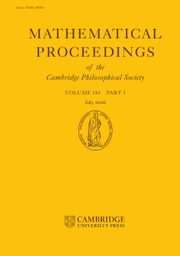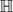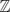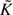Skip to main content Accessibility help
Home
Hostname: page-component-78dcdb465f-6zfdk Total loading time: 3.594 Render date: 2021-04-18T15:57:09.273Z Has data issue: true Feature Flags: { "shouldUseShareProductTool": true, "shouldUseHypothesis": true, "isUnsiloEnabled": true, "metricsAbstractViews": false, "figures": false, "newCiteModal": false, "newCitedByModal": true }Mathematical Proceedings of the Cambridge Philosophical Society

# Lower algebraic K-theory of certain reflection groups

Published online by Cambridge University Press:  20 November 2009

Corresponding
E-mail address:

## Abstract

For P3 a finite volume geodesic polyhedron, with the property that all interior angles between incident faces are of the form π/mij (mij ≥ 2 an integer), there is a naturally associated Coxeter group ΓP. Furthermore, this Coxeter group is a lattice inside the semi-simple Lie group O+(3, 1) = Isom(3), with fundamental domain the original polyhedron P. In this paper, we provide a procedure for computing the lower algebraic K-theory of the integral group ring of such groups ΓP in terms of the geometry of the polyhedron P. As an ingredient in the computation, we explicitly calculate the K−1 and Wh of the groups Dn and Dn ×3, and we also summarize what is known about the0.

Type
Research Article
Information
Copyright
Copyright © Cambridge Philosophical Society 2009

## Access options

Get access to the full version of this content by using one of the access options below.

## References

[An]Andreev, E. M.Convex polyhedra of finite volume in Lobačevskiĭ space. Math. USSR-Sb. 12 (1970), 255259.CrossRefGoogle Scholar
[Ba1]Bass, H.The Dirichlet unit theorem, induced characters and Whitehead groups of finite groups. Topology 4 (1965), 391410.CrossRefGoogle Scholar
[Ba2]Bass, H.Algebraic K-theory (W. A. Benjamin, 1968).Google Scholar
[BFJP]Berkove, E., Farrell, F. T., Juan-Pineda, D. and Pearson, K.The Farrell-Jones isomorphism conjecture for finite covolume hyperbolic actions and the algebraic K-theory of Bianchi groups. Trans. Amer. Math. Soc. 352 (2000), 56895702.CrossRefGoogle Scholar
[BB]Bley, W. and Boltje, R. Computation of locally free class groups, in Algorithmic number theory (Lect. Notes in Comput. Sci., 4076), 7286 (Springer, 2006).CrossRefGoogle Scholar
[C]Carter, D.Lower K-theory of finite groups. Comm. Algebra 8 (1980), 19271937.CrossRefGoogle Scholar
[CR1]Curtis, C. and Reiner, I.Methods of Representation Theory, Vol. I (John Wiley & Sons, Inc., 1990), xxiv+819 pp.Google Scholar
[CR2]Curtis, C. and Reiner, I.Methods of Representation Theory, Vol. II (John Wiley & Sons, Inc., 1987), xviii+951 pp.Google Scholar
[D]Davis, J. Some remarks on Nil-groups in algebraic K-theory, preprint available at arXiv:0803.1641.Google Scholar
[DKR]Davis, J., Khan, Q. and Ranicki, A. Algebraic K-theory over the infinite dihedral group, preprint available at arXiv:0803.1639.Google Scholar
[DQR]Davis, J., Quinn, F. and Reich, H. Algebraic K-theory of virtually cyclic groups, in preparation.Google Scholar
[Da]Davis, M.The Geometry and Topology of Coxeter Groups (Princeton University Press, 2008), xiv+584 pp.Google Scholar
[De]Deodhar, V. V.On the root system of a Coxeter group. Comm. Algebra 10 (1982), 611630.CrossRefGoogle Scholar
[EM1]Endó, S. and Miyata, T.On the projective class group of finite groups. Osaka J. Math. 13 (1976), 109122.Google Scholar
[EM2]Endó, S. and Miyata, T.On the class groups of dihedral groups. J. Algebra 63 (1980), 548573.CrossRefGoogle Scholar
[FJ1]Farrell, F. T. and Jones, L. E.Isomorphism conjectures in algebraic K-theory. J. Amer. Math. Soc. 6 (1993), 249297.Google Scholar
[FJ2]Farrell, F. T. and Jones, L. E.The lower algebraic K-theory of virtually infinite cyclic groups. K-theory 9 (1995), 1330.CrossRefGoogle Scholar
[FKW]Fröhlich, A., Keating, M. E. and Wilson, S. M. J.The class groups of quaternion and dihedral 2-groups. Mathematika 21 (1974), 6471.CrossRefGoogle Scholar
[GRU]Galovich, S., Reiner, I. and Ullom, S.Class groups for integral representations of metacyclic groups. Mathematika 19 (1972), 105111.CrossRefGoogle Scholar
[J]Jacobinski, H.Genera and decompositions of lattices over orders. Acta Math. 121 (1968), 129.CrossRefGoogle Scholar
[JKRT]Johnson, N. W., Ratcliffe, J. G., Kellerhals, R. and Tschantz, S. T.The size of a hyperbolic Coxeter simplex. Transform. Groups 4 (1999), 329353.CrossRefGoogle Scholar
[K]Keating, M. E.Class groups of metacyclic groups of order pr q, p a regular prime. Mathematika 21 (1974), 9095.CrossRefGoogle Scholar
[LO1]Lafont, J.-F. and Ortiz, I. J.Relative hyperbolicity, classifying spaces and lower algebraic K-theory. Topology 46 (2007), 527553.CrossRefGoogle Scholar
[LO2]Lafont, J.-F. and Ortiz, I. J.Lower algebraic K-theory of hyperbolic 3-simplex reflection groups. Comment. Math. Helv. 84 (2009), 297337.CrossRefGoogle Scholar
[LO3]Lafont, J.-F. and Ortiz, I. J.Relating the Farrell Nil-groups to the Waldhausen Nil-groups. Forum Math. 20 (2008), 445455.CrossRefGoogle Scholar
[Le]Lemmermeyer, F.Ideal class groups of cyclotomic number fields, II. Acta Arith. 84 (1998), 5970.CrossRefGoogle Scholar
[Li]van der Linden, F. J.Class number computations of real abelian number fields. Math. Comp. 39 (1982), 693707.CrossRefGoogle Scholar
[Lu]Lück, W.Survey on classifying spaces for families of subgroups, in “Infinite groups: geometric, combinatorial and dynamical aspects”. Progr. Math. 248 (2005), 269322.CrossRefGoogle Scholar
[LR]Lück, W. and Reich, H.The Baum-Connes and the Farrell-Jones conjectures in K- and L-theory, in Handbook of K-theory, Vol. 2, 703842 (Springer-Verlag, 2005).Google Scholar
[LW]Lück, W. and Weiermann, M. On the classifying space of the family of virtually cyclic subgroups, to appear in Pure Appl. Math. Q.Google Scholar
[Ma1]Magurn, B.SK 1 of dihedral groups. J. Algebra 51 (1978), 399415. Erratum to SK1 of dihedral groups. J. Algebra 55 (1978), 545–546.CrossRefGoogle Scholar
[Ma2]Magurn, B.Whitehead groups of some hyperelementary groups. J. London Math. Soc. 21 (1980), 176188.CrossRefGoogle Scholar
[Mi]Milnor, J.Whitehead torsion. Bull. Amer. Math. Soc. 72 (1966), 358426.CrossRefGoogle Scholar
[Ol]Oliver, R.Whitehead groups of finite groups. London Math. Soc. Lecture Note Series 132 (Cambridge University Press, 1988), viii+349 pp.CrossRefGoogle Scholar
[OT]Oliver, R. and Taylor, L. R.Logarithmic descriptions of Whitehead groups and class groups for p-groups. Mem. Amer. Math. Soc. 76 (1988), no. 392, vi+97 pp.Google Scholar
[Or]Ortiz, I. J.The lower algebraic K-theory of Γ3. K-theory 32 (2004), 331355.CrossRefGoogle Scholar
[Pe]Pearson, K.Algebraic K-theory of two dimensional crystallographic groups. K-theory 14 (1998), 265280.CrossRefGoogle Scholar
[Qu]Quinn, F.Ends of maps II. Invent. Math. 68 (1982), 353424.CrossRefGoogle Scholar
[R]Ratcliffe, J. G.Foundations of hyperbolic manifolds. Graduate Texts in Mathematics 149 (Springer-Verlag, 1994).CrossRefGoogle Scholar
[Re]Reiner, I.Maximal orders. Corrected reprint of the 1975 original. With a foreword by Taylor, M. J.London Math. Soc. Monogra. N. S. 28 (The Clarendon Press, Oxford University Press, 2003), xiv+395 pp.Google Scholar
[RU1]Reiner, I. and Ullom, S. Remarks on class groups of integral group rings, in “Symposia Mathematica. Vol. XIII (Convegno di Gruppi e loro Rappresentazioni, INDAM, Rome, 1972),” 501516. (Academic Press, 1974).Google Scholar
[RU2]Reiner, I. and Ullom, S.A Mayer-Vietoris sequence for class groups. J. Algebra 31 (1974), 305342.CrossRefGoogle Scholar
[RHD]Roeder, R. K. W., Hubbard, J. H. and Dunbar, W. D.Andreev's Theorem on hyperbolic polyhedra. Ann. l'Inst. Fourier 57 (2007), 825882.CrossRefGoogle Scholar
[Se1]Serre, J.-P.Local fields. Graduate Texts in Mathematics 67 (Springer-Verlag, 1979), viii+241 pp.CrossRefGoogle Scholar
[Se2]Serre, J.-P.Trees (Springer-Verlag, 1980).CrossRefGoogle Scholar
[St]Steinitz, E. Polyeder und Raumeinteilungen in Encyclopädie der mathematischen Wissenschaften Band 3 (Geometrie), Teil 3AB12 (1922), 1–139.Google Scholar
[Sw]Swan, R. G.K-theory of finite groups and orders. Lecture Notes in Math. 149. (Springer-Verlag, 1970), iv+237 pp.Google Scholar
[T]Taylor, M. J.Locally free classgroups of groups of prime power order. J. Algebra 50 (1978), 463487.CrossRefGoogle Scholar
[Wag]Wagstaff, S. S. Jr., The irregular primes to 125000. Math. Comp. 32 (1978), 583591.Google Scholar
[Wa]Wall, C. T. C.Norms of units in group rings. Proc. London Math. Soc. 29 (1974), 593632.CrossRefGoogle Scholar
[Wd]Waldhausen, F.Algebraic K-theory of generalized free product I, II. Ann. of Math. 108 (1978), 135256.CrossRefGoogle Scholar
[Wh]Whitney, H.Congruent graphs and the connectivity of graphs. Ameri. J. Math. 54 (1932), 150168.CrossRefGoogle Scholar
[We]Weibel, C.NK 0 and NK 1 of the groups C 4 and D 4. Comment. Math. Helv. 84 (2009), 339349.CrossRefGoogle Scholar
[Z]Ziegler, G. M.Lectures on polytopes. Graduate Texts in Mathematics 152 (Springer-Verlag, 1995), ix+370 pp.CrossRefGoogle Scholar

### Full text views

Full text views reflects PDF downloads, PDFs sent to Google Drive, Dropbox and Kindle and HTML full text views.

Total number of HTML views: 0
Total number of PDF views: 33 *
View data table for this chart

* Views captured on Cambridge Core between September 2016 - 18th April 2021. This data will be updated every 24 hours.

# Send article to Kindle

To send this article to your Kindle, first ensure no-reply@cambridge.org is added to your Approved Personal Document E-mail List under your Personal Document Settings on the Manage Your Content and Devices page of your Amazon account. Then enter the ‘name’ part of your Kindle email address below. Find out more about sending to your Kindle. Find out more about sending to your Kindle.

Note you can select to send to either the @free.kindle.com or @kindle.com variations. ‘@free.kindle.com’ emails are free but can only be sent to your device when it is connected to wi-fi. ‘@kindle.com’ emails can be delivered even when you are not connected to wi-fi, but note that service fees apply.

Find out more about the Kindle Personal Document Service.

Lower algebraic K-theory of certain reflection groups
Available formats
×

# Send article to Dropbox

To send this article to your Dropbox account, please select one or more formats and confirm that you agree to abide by our usage policies. If this is the first time you use this feature, you will be asked to authorise Cambridge Core to connect with your <service> account. Find out more about sending content to Dropbox.

Lower algebraic K-theory of certain reflection groups
Available formats
×

# Send article to Google Drive

To send this article to your Google Drive account, please select one or more formats and confirm that you agree to abide by our usage policies. If this is the first time you use this feature, you will be asked to authorise Cambridge Core to connect with your <service> account. Find out more about sending content to Google Drive.

Lower algebraic K-theory of certain reflection groups
Available formats
×
×

#### Conflicting interests

Do you have any conflicting interests? *